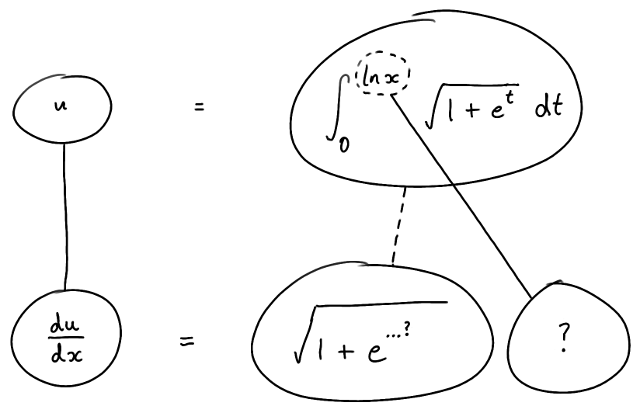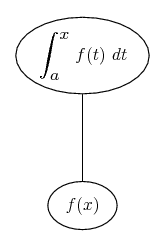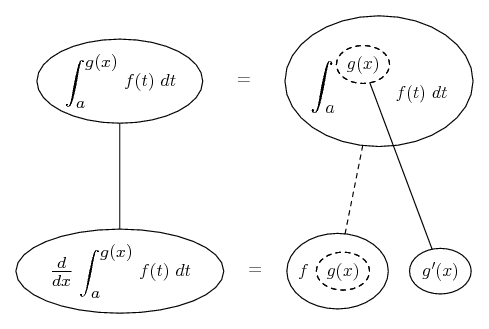# Thread: find y'(x) and y''(x) when y is defined with an integral

1. ## find y'(x) and y''(x) when y is defined with an integral

For x > 0, define $\displaystyle y(x) := x$ $\displaystyle \int_0^{log x} \! \sqrt{1 + e^t} dt -(2/3)(1 + x)^{3/2}$
Calculate $\displaystyle y'(x) := dy/dx$
and
y''(x) := $\displaystyle d^2y/dx^2$

2. You'll need the Leibniz Rule, the product rule, and the chain rule to differentiate your y(x). What do you get?

3. Since there is no x inside the integral, strictly speaking you don't need the full "Leibniz rule", just the "Fundamental theorem of Calculus" and the chain rule.

4. Just in case a picture helps...... where (key in spoiler) ...

Spoiler:... is the chain rule. Straight continuous lines differentiate downwards (integrate up) with respect to the main variable (in this case x), and the straight dashed line similarly but with respect to the dashed balloon expression (the inner function of the composite which is subject to the chain rule).

Then, combining this with...... the Fundamental Theorem of Calculus, we have..._________________________________________

Don't integrate - balloontegrate!

Balloon Calculus; standard integrals, derivatives and methods

Balloon Calculus Drawing with LaTeX and Asymptote!

#### Search Tags

defined, integral